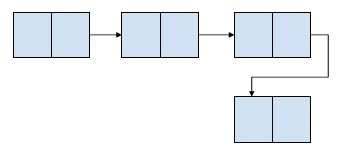# Print alternate nodes of a linked list using recursion in C++

A linked list is a linear data structure that stores the element in non-contiguous memory locations. Every element contains a pointer to the next element of the linked list.

ExampleIn this problem, we are given a linked list and we need to print the elements of this linked list but only alternate elements are to be printed. Let’s take an example to understand the problem better,

Input : 2 -> 4 -> 1 -> 67 -> 48 -> 90
Output : 2 -> 1 -> 48

Explanation − We will print alternate elements on the linked list. So first, third and fifth elements are printed.

We will use a flag element that will initially be 0 and will be increased at every iteration that prints element otherwise decrease it and we will print the node value when the flag is 0.

## Example

Live Demo

#include <stdio.h>
#include <stdlib.h>
struct Node {
int data;
struct Node* next;
};
int flag = 0;
if (flag == 0){
flag = 1;
}
else
flag = 0;
}
}
void insertNode(struct Node** head_ref, int new_data){
struct Node* new_node = (struct Node*)malloc(sizeof(struct Node));
new_node->data = new_data;
}
int main(){
}
71  98  23